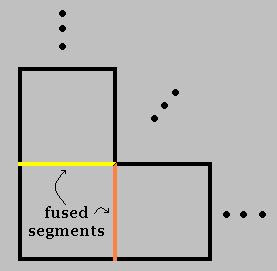May 25, 2007

Visualizing the fourth dimension, Part 1

Via Uncertain Principles, here is a neat PowerPoint presentation on visualizing a hypercube. I don't promise that any of this series will be easy reading because 1) we're talking about weird stuff, and 2) I'm not getting paid for this, so it may not be as perfectly polished as a textbook would be. But who said visualizing a 4-D object would be easy?

Before getting to how to visualize a hypercube, let's note that this is not the same thing as "visualizing in four dimensions." Allow me to rain on the parade as follows -- see picture below: by visualing a 2-D cube (that is, a square), we can proceed to visualize all of 2-D space by fusing together the (n-1) dimensional "faces" of a square with those of another square (that is, the line segments that make up the perimeter). Say we fused the right side of square A with the left side of square B, making two squares side-by-side; we could do this infinitely in the left-right dimension. Then we could fuse the top of square A with the bottom of a third square C, and again stack as many others as we wanted in the up-down dimension. In this way, we'd fill out all of a 2-D coordinate system, like everyday graph paper.To visualize all of 3-D space -- see picture below -- we'd start with the (n-1) dimensional faces of a cube (that is, the squares making up the surface) and fuse them just as we did before. Since there are 3 dimensions we can travel in, we'd fuse the square sides of a bunch of cubes left or right, up or down, and toward or away from our viewpoint, filling up 3-D space. Note that the picture only shows this in one dimension to minimize clutter -- the top of the left-hand cube would be fused with the bottom of a cube on top of it, and the back of the left-hand cube would be fused with the front of a cube behind it.The problem comes when we try to do this in 4-D: the "faces" of a hypercube are (n-1) dimensional cubes -- that is, 3-D cubes -- and we have to fuse these between adjacent hypercubes in four dimensions. You can't do this in 2-D, anymore than you could visualize 3-D space using only 1-D, so you'd need to build a 3-D model. The trouble is that, unlike fusing a bunch of square faces in a 2-D picture to imagine what 3-D would look like, which you can do with a special kind of graph paper, building a 3-D model of a 4-D coordinate system in the same way would require too much effort to be worth it, unless you were a real geek. So, the best we can do without really slaving away is to visualize just a 4-D (hyper)cube -- as if we could only visualize one cube in 3-D or one square on 2-D graph paper. Pretty myopic, but do-able.

In Part 2, we'll take a necessary detour through some graph theory, which will greatly aid us in thinking about how to go from 3-D to 4-D, which we'll do later on still.